# Average Acceleration and Instantaneous Acceleration

Average Acceleration

For any change in velocity either in its magnitude or direction or both, acceleration must be present. Without acceleration neither magnitude nor direction of velocity can be changed.

When a particles velocity changes, the particle is said to undergo acceleration.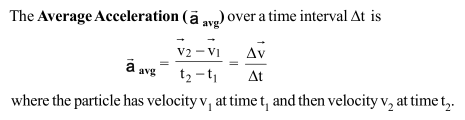Instantaneous Acceleration

The Instantaneous Acceleration is derivative of the velocity with respect to time.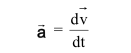In words, acceleration of a particle at any instant is the rate at which its velocity is changing at that instant.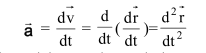In words, the acceleration of a particle at any instant is second derivative of its position vector with respect to time.

Acceleration has both magnitude and direction. For motion on a straight line its algebraic sign represents its direction on an axis just as for displacement and velocity; that is, acceleration with a positive value is in the positive direction of an axis, and acceleration with a negative value is in the negative direction.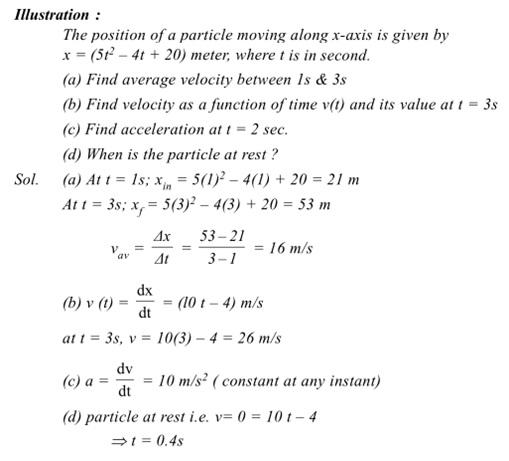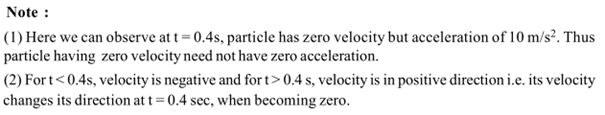Post By : Divya SIngh 06 Feb, 2020 2572 views Physics